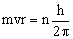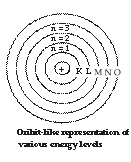# Chemistry Assignment Help with Bohr's Model

## 3.6 Bohr's Model

Niel Bohr applied the concept of Max Planck to the problem of revolving electron around hydrogen nucleus and gave the following main points of what is now known as Bohr's theory of atomic structure. The various postulates of Bohr's theory are as follow.

(i) That within the atom an electron can move in certain specific orbits without radiating out any energy. Such orbits are termed as stationary orbits. These orbits are numbered as 1, 2, 3, 4 etc., or K, L, M, N, etc., starting from the nucleus.(ii) The mathematical condition for stationary orbits is that the angular momentum for the moving electron is an integral multiple of h/2p, where h is the Planck's constant.

orwhere mvr denotes the angular momentum and n is called principal quantum number and is equal to 1, 2, 3…(iii)Even though an electron in privileged orbit is constantly accelerated, it does not radiate energy. Thus, the total energy of an electron in stationary state remains constant.

(iv)When an electron gets energy, it will go to higher energy orbits, Similarly in the reverse process, the excited electron jumps down to lower energy level by emitting absorbed energy in the form of radiations of suitable wavelength.

The frequency of this radiations (n) is given by the difference in energy between the initial and final orbits.

E2 – E1 = hv …(1)

Where E1 and E2 are the energies of electron in two stationary states (E2 > E1), h the Planck's constant and n is the corresponding frequency of radiation.

Equation (1) is known as Einstein's frequency condition.

With the help of Bohr’s theory, we can calculate.

(1) radius of the orbit (rn) in which electron is revolving around the nucleus.

(2) energy of e– (En) in an orbit.

(3) velocity of e– (vn) in an orbit.

(4) Frequency of e¯ revolving around the nucleus### Email Based Assignment Help in Bohr's Model

Bohr's Model is a topic in chemistry atomic structure. If you want Assignment Help homework help in Bohr's model our online tutors is available for you.